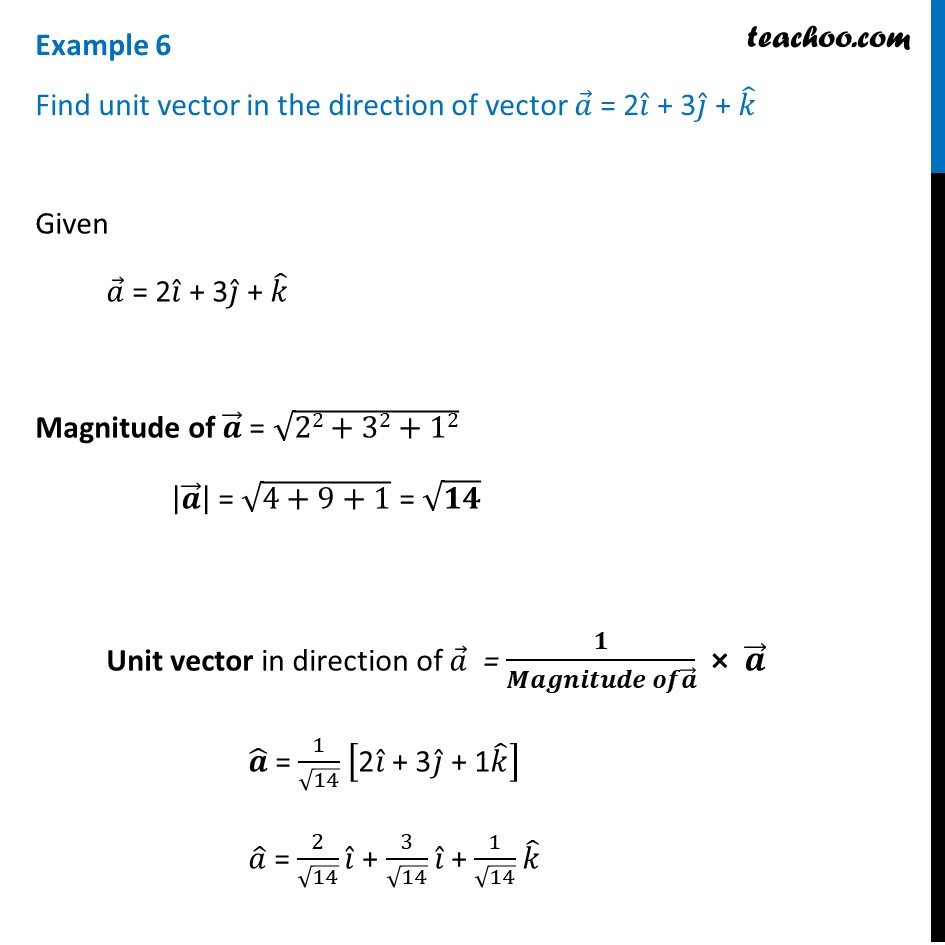Examples

Chapter 10 Class 12 Vector Algebra
Serial order wiseLearn in your speed, with individual attention - Teachoo Maths 1-on-1 Class

### Transcript

Example 6 Find unit vector in the direction of vector 𝑎 ⃗ = 2𝑖 ̂ + 3𝑗 ̂ + 𝑘 ̂ Given 𝑎 ⃗ = 2𝑖 ̂ + 3𝑗 ̂ + 𝑘 ̂ Magnitude of 𝒂 ⃗ = √(22+32+12) |𝒂 ⃗ | = √(4+9+1) = √𝟏𝟒 Unit vector in direction of 𝑎 ⃗ = 𝟏/(𝑴𝒂𝒈𝒏𝒊𝒕𝒖𝒅𝒆 𝒐𝒇𝒂 ⃗ ) × 𝒂 ⃗ 𝒂 ̂ = 1/√14 ["2" 𝑖 ̂" + 3" 𝑗 ̂" + 1" 𝑘 ̂ ] 𝑎 ̂ = 2/√14 𝑖 ̂ + 3/√14 𝑖 ̂ + 1/√14 𝑘 ̂